# Abstract

A reliability-based design optimization (RBDO) incorporates a probabilistic analysis with an optimization technique to find a best design within a reliable design space. However, the computational cost of an RBDO task is often expensive compared to a deterministic optimization, which is mainly due to the reliability analysis performed inside the optimization loop. Theoretically, the reliability of a given design point can be obtained through a multidimensional integration. Integration with multiple variables over the safety domain is, unfortunately, formidable in most cases. Monte- Carlo simulation (MCS) is often used to solve this difficulty. However, the inherit statistic uncertainty associated with MCS sometimes causes an unstable RBDO solution. To avoid this unstable solution, this study transforms a multi-variable constraint into a single variable constraint using an exponential function with a polynomial coefficient (EPM). The adaptive Gauss-Kronrod quadrature is used to compute the constraint reliability. The calculated reliability and its derivative are incorporated with an optimizer such as sequential quadratic programming (SQP) or most probable point particle swarm optimization (MPP-based PSO) to conduct the RBDO task. To ensure the design accuracy, the stability of the RBDO algorithm with respect to the initial point is investigated through several numerical examples.

Reliability; Optimization; EPM; RBDO; MPP-based PSO

A single loop reliability-based design optimization using EPM and MPP-based PSO

Kuo-Wei Liao* * Email: kliao@mail.ntust.edu.tw ; Gautama Ivan

Department of Construction Engineering, National Taiwan University of Science and Technology, No.43, Sec. 4, Keelung Rd., Taipei, Taiwan, 106

ABSTRACT

A reliability-based design optimization (RBDO) incorporates a probabilistic analysis with an optimization technique to find a best design within a reliable design space. However, the computational cost of an RBDO task is often expensive compared to a deterministic optimization, which is mainly due to the reliability analysis performed inside the optimization loop. Theoretically, the reliability of a given design point can be obtained through a multidimensional integration. Integration with multiple variables over the safety domain is, unfortunately, formidable in most cases. Monte- Carlo simulation (MCS) is often used to solve this difficulty. However, the inherit statistic uncertainty associated with MCS sometimes causes an unstable RBDO solution. To avoid this unstable solution, this study transforms a multi-variable constraint into a single variable constraint using an exponential function with a polynomial coefficient (EPM). The adaptive Gauss-Kronrod quadrature is used to compute the constraint reliability. The calculated reliability and its derivative are incorporated with an optimizer such as sequential quadratic programming (SQP) or most probable point particle swarm optimization (MPP-based PSO) to conduct the RBDO task. To ensure the design accuracy, the stability of the RBDO algorithm with respect to the initial point is investigated through several numerical examples.

Keywords: Reliability, Optimization, EPM, RBDO, MPP-based PSO

1 INTRODUCTION

A deterministic optimization uses a single value to represent a physical quantity in a calculation. It is known that uncertainties in an engineering design problem are often inevitable. One can utilize the concept of a safety factor to take uncertainties into consideration. The safety factor approach, however, does not identify the more critical design parameters because all uncertainties are represented by one factor throughout the design. Another approach such as the Load and Resistance Factor Design (LRFD), which is used for designing steel structures, also has a similar drawback. In LRFD, different factors are multiplied with each load type and resistance, such as the yielding stress. For example, for the load types of dead and live loads, the factors could be 1.2 and 1.6, respectively. For a structure design using LRFD, the factored resistance of that structure should be larger than the demand calculated from the factored loads. LRFD considers each design parameter inequitably through the assigned factors. However, a LRFD structure does not distribute the material in an optimal way because the same type of loads applied at different locations are still considered as identical in importance in LRFD, which is not true in most cases. A reliability-based design optimization (RBDO) takes an important step beyond a deterministic design optimization by not only characterizing the probabilistic aspects of the engineering problem but also identifying the relative importance of each design parameter. An RBDO can deliver an optimal design that satisfies the probabilistic constraints. For example, Beck and Verzenhassi (2008) used reliability-based risk optimization to find the optimum balance between safety and economy in the presence of uncertainty. Traditionally, an RBDO is conducted through a double-loop approach (known also as a two-level approach) in which the inner loop computes the constraint reliability and the outer loop conducts the optimization. The drawback of this approach is that its computational cost is usually expensive (Liao and Ha 2008; Ramu et al. 2004). Many algorithms have been proposed to lessen this computational burden. Aoues and Chateauneuf (2010), Li et al. (2010) and Liang et al. (2007) have categorized these algorithms into two groups: the single-loop approach (known also as the mono-level approach) and the decoupled approach (known also as the decomposed approach).

The decoupled approach assumes that the reliability levels of different designs can be approximately estimated by a constant number or evaluated in a deterministic approach. That is, an equivalent deterministic constraint is built to replace a probability constraint. In this case, the evaluation of the constraint reliability in the inner loop is eliminated, and an RBDO problem becomes an equivalent deterministic optimization problem. However, the reliability levels of different designs are not identical, and the decoupled approach often suggests to find another equivalent deterministic constraint determined by a design closer to the optimal point. After updating the reliability estimation to be more accurate, the equivalent deterministic optimization is reformulated and used to find the optimal solution of the next iteration. These processes are repeated until the optimal solutions are converged.

Examples of using the decoupled approach are briefly introduced as follows. Tu et al. (2001) and Zou and Mahadevan (2006) decoupled an RBDO problem through revising the constraints. In their approaches, the constraints were approximated by using Taylor expansion, which was performed repeatedly to increase the accuracy of the reliability approximation. Li et al. (2010) and Liao and Lu (2012) modified the right hand side (RHS) values of the constraint functions to find the equivalent-deterministic constraint functions. Similarly, the RHS values must be updated sequentially to capture the reliability performance near the optimal design point. Du and Chen proposed the Sequential Optimization and Reliability Assessment (SORA) method, in which the inverse most probable point (IMPP) was used to replace the random variables and to decouple the reliability analysis and optimization. The IMPP was re-evaluated at the optimal solution from a deterministic optimization to provide a more accurate reliability approximation for the use in the next deterministic optimization. Liao and Lu (2012) used a set of deterministic variables, called auxiliary design points, to replace the random variables to form a deterministic optimization that is equivalent to the RBDO problem. Similarly, the deterministic optimization and the calculation of finding the auxiliary design points were conducted iteratively until the solutions converged.

Another common strategy to lessen the computational burden of the double-loop approach is the single-loop approach. Chen et al. (1997) proposed a single-loop-single-vector (SLSV) to reduce the computational cost. The SLSV evaluated the reliability level at each design point during optimization. The reliability level at each design point was computed by using an approximated MPP. If a limit state function is highly nonlinear, the SLSV may not be able to deliver an optimized solution. Liang et al. (2007) extended the single-loop approach for an RBDO problem with series system reliability. Kaymaz and Marti (2007) proposed a mono-level RBDO approach, which was formulated by means of the necessary optimality conditions of the MPP. This approach, however, increases the number of design variables and increases the computational cost (Liang et al. 2007). Shan and Wang (2008) proposed another single-loop approach in which a reliable design space (RDS) was built, and an RBDO problem became a deterministic optimization problem constrained by RDS rather than the deterministic feasible space.

The current study uses the exponential function with the polynomial coefficient method (EPM) to represent the probability density function (PDF) of a limit state function. That is, a multivariable constraint is converted into a function with only one variable (Er 1998). In this way, the constraint reliability can be estimated by any existing numerical integration methods or simulation techniques such as Markov-Chain Monte-Carlo (MCMC) simulation. The calculated reliability or its derivative is incorporated with the sequential quadratic programming (SQP) or the most probable point particle swarm optimization (MPP-based PSO) algorithm to conduct an RBDO task. Because the reliability evaluation is conducted at each design point during optimization, similar to the single-loop approach, only one optimization task is needed for the proposed algorithm. To ensure the accuracies of the proposed RBDO approaches, the sensitivity of the proposed RBDO approaches with respect to the initial point is investigated. Several numerical examples are used to demonstrate the stability and accuracy of the proposed RBDO algorithm.

2 FORMULATION OF AN RBDO PROBLEM

A general formulation of an RBDO model is described by Equation (1), where f is the design objective, D is the vector of design variable, P is the vector of the random design variable to represent the uncertainty in the design variable, X is the vector of the random design parameter, Prob. stands for the probability, Gi is the ith constraint function, αi is the reliability requirement for the ith constraint and m denotes the number of constraints:In deterministic optimization, uncertainties in the design variables/parameters are ignored. Random design variables/parameters remain constant during optimization, and the constraint function is defined as Gi = (D, P, X) < 0. In this case, the reliability of the optimal design is often low because the process fails to capture uncertainties in the design variables/parameters. As shown in Equation (1), a typical RBDO is a nested double-loop approach, where the outer loop conducts the optimization and the inner loop considers the probabilistic analysis.

3 DESCRIPTION OF THE PROPOSED RBDO ALGORITHM

This study uses the EPM to convert a multiple-variable constraint into a single-variable constraint. After obtaining the single-variable constraint, this study uses an existing numerical integration technique called adaptive Gauss-Kronrod quadrature (GKQ) to evaluate the reliability of a given design. Results of EPM are incorporated with two optimizers, which are SQP and MPP- based PSO, to perform an RBDO task. If the SQP, rather than a MPP-based PSO, is used as the optimizer, the reliability gradient is computed by the finite difference method. A detailed description of the proposed algorithm is provided below.

3.1 Reliability analysis

For time-independent reliability analysis, the failure probability of a given design can be written as Equation (2).where fx() is the PDF in X, the vector of basic variables in n-space. The "failure domain" D is defined by one or more constraint functions Gi(X) < 0, i = 1, 2, ..., m. The integral in Equation (2) above is in general very complicated to calculate. Approximate methods for estimating the failure probability have been proposed, such as first order reliability analysis (FORM) and Monte-Carlo Simulation (MCS). FORM computes the failure probability through an optimization procedure. The objective is to minimize the distance between the origin and the MPP in the reduced space, and the constraint is that the MPP must be on the limit state function. The obtained distance is called reliability index (β), and, the failure probability can be easily calculated from β. MCS is considered as a simulation approach in which the basic variables are generated based on their PDFs and the limit state function is repeatedly evaluated using the generated variables. If the failure probability is very small, a large sample size is often required for the MCS approach.

If the integral in Equation (2) can be reduced to a one-dimensional problem, the evaluation of the reliability will not be a burden. Thus, this study adopted the EPM approach (Er 1998) to convert the integral, described in Equation (2), from a multiple space into a single space. EPM assumes that the PDF of a random variable X can be approximated by an exponential function with a polynomial function. That is, the coefficient of the exponential function is a polynomial function. The coefficients in the polynomial function can be solved through a set of simultaneous linear algebraic equations using the moments of the random variables, which can be solved with a little computational effort. EPM is briefly described below.

Suppose that the exact PDF of random variable X is denoted as px (x) and can be approximated as qx(x), as described in Equation (3).where c is normalizing constant and is determined by Equation (4).where Q(x) is a polynomial function that is defined in Equation (5).in which ai, i = 1, 2, ∙∙∙ are the coefficients of the polynomial function to be determined. In practice, the truncated form of the polynomial Qn(x) and qn(x) may be used as described in Equation (6).where αand βare two constants. By setting the residual error, δ(x), as described in Equation (7), equal to zero, one can obtain Equation (8) to solve the coefficients (ai) in Qn(x).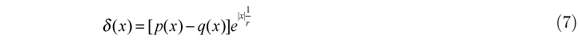where r is a large positive integer.where the variable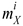(i = 1, 2, ∙∙∙) is defined as E[Xi] = ∫ (x)xi dx. Equation (8) can be written in the following matrix form, which is a set of n simultaneous algebraic equations.As mentioned earlier, once the limit state function is converted into a function with a single random variable using EPM, GKQ is used to calculate the failure probability.

3.2 Optimization: SQP and MPP-based PSO

Optimization methods using mathematical programming such as SQP are very efficient. However, the stability of applying SQP in an RBDO problem has not been thoroughly investigated. To verify the stability, this study uses SQP to repeatedly solve an identical RBDO problem by using different initial points. The results indicate that there is room to improve the stability of using SQP. For this reason, this study adopts another population-based optimization method (PSO) to perform an RBDO problem. The proposed MPP-based PSO is briefly described below.

PSO mimics a natural movement of organisms in a bird flock or fish school and is a stochastic global optimization method (Parsopoulos and Vrahatis 2002). PSO, unlike the gradient-based optimization algorithm, finds the optimal point from multiple starting points or particles. Each starting point/particle has its own search path during optimization. That is, a sequence of points is generated for each starting particle, and the collection of these points is called a population. Many variations have been proposed for the PSO; the following describes the detailed PSO used in current study.

A new particle is generated by using Equation (10), as shown below.where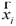(t + 1) denotes the position of the ith particle in the next iteration,(t) denotes the position of the ith particle in the current iteration and(t + 1) denotes the velocity of the ith particle in the current iteration. The position of a particle represents the design values of that particle. The velocity of the ith particle is determined by Equation (11), as shown below.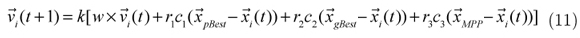where k is the constriction factor that is used to prevent oscillation of the design variable, w is the inertia factor,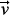i(t) is the velocity of at previous iteration, ri(i = 1-3) are random numbers between 0 and 1, and c1, c2 and c3 are the cognition factor, the social factor and the acceleration factor, respectively.pBest is the particle position with the minimum objective value in the ith population,gBest is the particle position with the minimum objective value among all populations, andMPP is the MPP of the current position (i (t)). Ideally, the optimal solution should stay on the reliability constraints. However, the standard PSO does not make any effort to make this occur. Thus, (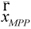-(t)) is added to the standard PSO algorithm to drive the search movement to the vicinity of the reliability constraints. Meanwhile, to prevent a particle from staying in the infeasible domain for a long time, this study places a particle at the probabilistic boundary when that particle is directed to the infeasible domain. A reliability analysis is needed to identify the location of a probabilistic boundary, which certainly affects the computational cost. To reduce the number of reliability analyses, a balance strategy is used, which is only to maintaingBest in the feasible domain. That is,pBet does not necessarily satisfy the reliability constraints. Conversely,gBest always meets the reliability requirement.

Four major factors--the previous velocity, the local best (pBest), the global best (gBest) and the MPP (MPP)--affect the current particle velocity. These four factors are controlled by the inertial weight (w), the cognition factor (c1), the social factor (c2) and the acceleration factor (c3). Apparently, the larger the cognition factor, the greater tendency that the particle will move towards the local best (pBest). A similar conclusion can be applied to the social factor and the acceleration factor. There are many existing algorithms proposed to determine the ci factors. In this study, ci are determined by a grid search, and they were 1.5, 1.7 and 1.0 for c1, c2 and c3, respectively. The inertia weight used in PSO provides a mechanism to control the exploration and exploitation abilities of the swarm. The inertia weight is calculated using Equation (12) as follows.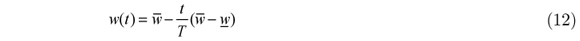where t is the current iteration number, T is the total number of iterations, and(=1) and w(=0) are the upper and lower bounds of the inertia weight, respectively. Equation (12) indicates that the inertia weight decreases as the iteration number increases. This technique moves a particle more smoothly and more gradually (exploration) at the beginning stage. In addition, Equation (12) allows a particle to settle into the optima (exploitation) at the ending stage.

In addition to the factors above, other issues that affect PSO are the number of particles/populations, the number of iterations, the position of the initial particles and the initial velocity of each particle. The numbers of particles/populations and iterations used in the current study are 30 and 80, respectively. The initial positions are often randomly determined. In this research, Latin Hypercube Sampling (LHS) is adopted to generate initial design values that are more scattered in the design domain.

4 NUMERICAL EXAMPLES

4.1 A cantilevered beam problem

The literature study from Liao and Ha (2008), Wu et al. (2001), Ramu et al. (2006) and Qu and Haftka (2004) is adopted as the first example to demonstrate the proposed RBDO algorithm. The objective of this example is to find the minimum weight or the minimum cross sectional area of a cantilevered beam as described in Equation (13) and Figure 1.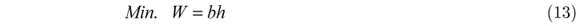The width (b) and height (h) of the beam are the design variables with lower and upper bounds of 0 and 10, respectively. The two loads (x, y) at the free end, the modulus of elasticity (E) and the yield strength (r) are the random parameters, and their statistics are provided in Table 1. The length of the cantilevered beam is 254 cm (100 inches). Two limit state functions are considered separately. In Case 1, the stress at the fixed end has to be less than the yield strength (r), and, in Case 2, the tip displacement has to be smaller than the allowable displacement (d0 = 7.724 cm or 2.2535 inches). The target reliability is set to 99.865% (i.e., β = 3) for both cases. For Case 1, the limit state function (Gs) is described by Equation (14):while, for Case 2, the limit state function (GD) is expressed by Equation (15):4.1.1 Case 1

Four different RBDO algorithms were used to solve this case: the double-loop approach using SQP as the optimizer and using FORM for reliability analysis (D-SQP-FORM), the double-loop approach using SQP as the optimizer and using MCS for reliability analysis (D-SQP-MCS), the single-loop approach using SQP as the optimizer and using EPM for reliability analysis (S-SQP- EPM) and the single-loop approach using PSO as the optimizer and using EPM for reliability analysis (S-PSO-EPM). Because the current problem does not have a local optimal point and the constraint is linear, the D-SQP-FORM should deliver an accurate and identical solution given different initial points. Thus, the solution of D-SQP-FORM was used as the benchmark design. To demonstrate this idea, 9 different values of 1, 2, 3,..., 9 for each design variable were chosen, resulting in 81 initial points that were used in the D-SQP-FORM approach. These 81 optimal values, as expected, are identical, and they are 9.520246 with b = 2.44599 and h = 3.892185. These results are similar to the results from Liao and Ha (2008), Wu et al. (2001), Ramu et al. (2006) and Qu and Haftka (2004), which are shown in Table 2.

Although MCS with sufficient sample size is often considered a suitable tool for reliability analysis, it is not an easy task to completely eliminate the effect of the inherit statistic uncertainties of MCS on an RBDO solution (Liao and Lu 2012). To investigate this effect, D-SQP-MCS was used to solve the current RBDO problem. The same 81 initial points were used for the D- SQP-MCS approach. For each initial point, the D-SQP-MCS method was performed twice. That is, this problem was analyzed 162 times. Ideally, the goal of an optimization algorithm is to deliver an identical and accurate solution given different initial points. However, an RBDO problem either has a local minimum or has a nonlinear constraint. In this case, it is very difficult to achieve this goal. Instead, if the difference between a solution and the "target solution" is less than 2% of the optimal solution, that solution is considered as a "reasonable solution" in current study. The "target solution" for the current problem is the solution from D-SQP-FORM in which the objective value is 9.5202. Based on this definition, Tables 3 and 4 shows that D-SQP- MCS only delivered 19 and 17 reasonable solutions (the number of green blocks), respectively. The sample size used in these analyses is 106. With this sample size, the effect of statistical uncertainty on an RBDO solution cannot be removed. To further investigate the influence of the statistical uncertainty, other sample sizes such as 103, 10 4 and 10 5 are used for MCS. The results are displayed in Table 5. The number of reasonable solutions did not significantly increase when a greater sample size was used.

Unlike the MCS, there is no statistic uncertainty in the reliability evaluation by using EPM incorporated with GKQ. It is of interest to investigate the sensitivity of the S-SQP-EMP solution with respect to the initial point. The results of S-SQP-EMP approach are displayed in Table 6. There were 36 reasonable solutions. Compared to the approach of D-SQP-MCS, the S-SQP- EMP approach is apparently less sensitive to the initial point. Thus, S-SQP-EMP is a more stable RBDO approach.

Another way to examine the effect of statistic uncertainty on the RBDO solution is to conduct an RBDO problem using the identical initial point (7, 9) several times. Table 7 displays the 20 solutions of the Case 1 problem using the D-SQP-MCS and S-SQP-EMP approaches. For the D-SQP-MCS, increasing sample size can effectively reduce the variation in solutions. The coefficient of variation (cov) in solutions decreased from 0.038 to 0.013. In addition, the RBDO solution is not significantly affected by statistic uncertainty when the sample size is greater than 103 . However, greater sample size does not always produce more reasonable solutions, as shown in Table 5. Thus, the capability of delivering a reasonable solution for D-SQP-MCS depends on both the sample size and location of initial point. As results shown in Tables 5 and 7, one can conclude that the impact of initial point is significantly. No deviation among the 20 S-SQP-EMP solutions is found. Thus, it is confirmed that S-SQP-EMP is a more stable RBDO algorithm than D-SQP-MCS.

Although S-SQP-EMP is more stable than D-SQP-MCS, the stability of S-SQP-EMP still needs to be improved. As shown in Table 6, there are still 45 unreasonable solutions when using the S-SQP-EMP approach. To investigate further, three arbitrary points, (2.45, 3.90), (2.0, 3.0) and (1.0, 2.0), are selected to perform the reliability analysis using FORM, MCS and EPM. Table 8 shows that, for the point (2.45, 3.90), EPM generally delivered more promising solutions compared to MCS. To be specific, EPM with three moments has a better reliability evaluation than MCS with a sample size of 107. MCS with a sample size that is less than 105 is more efficient than EPM. However, their evaluations were relatively inaccurate.

In the case of point (2.0, 3.0), only the D-SQP-MCS failed to deliver a reasonable solution (Tables 3, 4 and 6). Table 9 displays the failure probability and the gradient with respect to the horizontal force (x) for the approaches of FORM, MCS and EPM. For the failure probability, all three approaches were very close. Because a gradient-based optimizer (SQP) is used at this stage, it is worth examining the gradients of the three approaches at this point. The MCS gradient was apparently different than the gradients of the other two approaches. Thus, for this particular point, the inaccurate gradient information calculated by MCS may result in an unreasonable solution for the D-SQP-MCS approach.

Another point, (1.0, 2.0), was selected for a similar investigation. For this point, only the D- SQP-FORM can deliver a reasonable solution (Tables 3, 4 and 6). The failure probabilities obtained using FORM, MCS and EPM are displayed in Table 10. Although both MCS and EPM did not provide an accurate reliability, there was only slight difference compared to the reliability of FORM. The gradients calculated by FORM, MCS and EPM are also shown in Table 10. At this particular point, EPM provided accurate gradient information but incorrect reliability. MCS provided an approximated reliability but incorrect gradient information. Thus, both D-SQP- MCS and S-SQP-EPM failed to deliver an accurate RBDO solution.

Based on results found in Tables 8, 9 and 10, if the point is located near the "target solution" or in the feasible domain, the chance to deliver a reasonable solution is higher for the D-SQP- MCS and S-SQP-EPM approaches. However, if the point is located in the infeasible domain, the above two approaches often fail to deliver a reasonable solution due to an inaccurate evaluation of the reliability and the gradient. In addition, the statistic uncertainty has a significant impact on the stability of an RBDO solution, and thus D-SQP-MCS delivers a less reasonable solution than S-SQP-EPM.

EPM is generally a more efficient method for reliability evaluation compared to MCS. However, if the design point is located in the infeasible region, EPM combined with SQP often fails to find a good RBDO solution. In this case, this study suggests the use of another optimizer (PSO) to conduct the optimization to consistently deliver an optimal solution.

To prevent the particle from being trapped in a local minimum, a space-filling experiment design (i.e., the Latin hypercube) is used to generate the first generation of the particles in PSO. Because PSO is a population-based algorithm, S-PSO-EPM was performed 10 times to display the variation among solutions. Thirty particles and 80 iterations were used in PSO for the current study. The statistics of the S-PSO-EPM solutions are displayed in Table 11. The history of the objective value for 10 runs is shown in Figure 2. As shown in Figure 2, for most analysis repetitions, the alteration of the global best after the 40th iteration is very small. Compared with the "target solution" (9.520246), all of the results of the S-PSO-EPM analysis were within a range of less than 1% deviation. That is, all solutions can be considered as a reasonable solution, indicating that the PSO algorithm is perfectly integrated with the EPM. S-PSO-EPM was thus shown to be able to consistently deliver an optimal solution and thus is a stable RBDO algorithm.

4.1.2 Case 2

In contrast to the stress limit state problem considered in the first case, the second case is a nonlinear problem. In this situation, no analytical reliability analysis is available. To examine the stability of an RBDO algorithm, the optimal point (2.721, 3.392) from Liao and Ha (2008) is used as the target solution instead of using the D-SQP-FORM solution. Both S-SQP-EPM and D- SQP-MCS (with sample sizes of 103, 104, 105 and 106) were used to solve the current RBDO task using 81 identical initial points, as explained in the previous section. Similarly, D-SQP-MCS was conducted twice for each initial point. The summary of the number of reasonable solutions is displayed in Table 12. When the limit state function is nonlinear, S-SQP-EPM still delivers more reasonable solutions than D-SQP-MCS. The number of reasonable solutions is moderately affected by the sample size. The results of using S-PSO-EPM are provided in Table 13 and Figure 3. The solutions converged at approximately 30 iterations. In addition, all optimal points were a reasonable solution. For the case of the nonlinear limit state function, S-PSO-EPM was able to deliver a consistent RBDO solution among the different trials.

4.2 A three bar truss problem

The second example is a three bar truss problem, that is adopted from Thanedar and Kodiyalam (1992), displayed in Figure 4. The statistics of the random parameters are displayed in Table 14. The optimization formulation is shown below.where W is the total weight of the structure, A1 is the cross-sectional area for members 1 and 3 (inch2), A2 is the cross-sectional area for member 2 (inch2), Px (10000lbs) and Py (20000lbs) are the forces acted on the point 4 in the x and y directions, respectively, l is 10 inches, E is the elastic modulus (N(1.0x107, 0.12) with units of psi, and δa is the allowable displacement (N(0.005, 0.12)) with units of inches. The optimization goal is to minimize the total weight of the structure subjected to two deterministic forces. Two probabilistic displacement constraints (G1, G2) are formulated to ensure that the resulting displacements at point 4 in x and y directions meet the predefined reliability (β = 2). Another three deterministic stress constraints are formulated to ensure that the resulting compressive/tensile stresses for members 1, 2 and 3 are less than 5000 psi, 20,000 psi and 5000 psi, respectively.

Similar to the first example, this example was analyzed 81 times using different initial points for both the D-SQP-MCS and S-SQP-EPM approaches. The initial point is a pair (Ai, Aj), where Ai and Aj are values of 1, 2, 3,..., 9, resulting in 81 different points. Because the probability density function (PDF) is not provided in the literature study of Thanedar and Kodiyalam (1992), this study assumes that all random parameters follow a normal distribution, as shown in Table 14. The "target solution" is defined as the "best solution" among the 81 S-SQP-EPM solutions, and it is 21.112 with a design point of 6.31 and 3.265, as indicated in Table 15. The reliability analysis at the "target solution" was conducted, and the result is displayed in Table 15. It is clear that the "target solution" satisfied the reliability requirement. The number of reasonable solutions for D-SQP-MCS and S-SQP-EPM are provided in Table 16. The statistical uncertainty produced by the MCS results in a very unstable solution for the D-SQP-MCS approach. Although increasing the sample size can effectively reduce the variation in the number of reasonable solutions, it does not promise to have a greater number of reasonable solutions. S-SQP-EPM certainly dominated over D-SQP-MCS in terms of solution stability for any sample size.

The results of using S-PSO-EPM for the second problem are provided in Table 17 and Figure 5. The range for the 10 solutions was from 21.112 to 21.201. None of these solutions has a deviation that is larger than 2% from the "target solution" (21.112), indicating that S-PSO-EPM is a suitable and stable optimization algorithm.

4.3 A mathematical problem with multiple limit states

The mathematical formulation of this problem, adopted from Youn and Choi (2004), is provided in Equation (17).where D are the design variables, which are the mean values of the random design variables (P), in which P ~ N (D, 0.62). Gi is the ith constraint. The target reliability is 97.72% (β = 2) for all three constraints. In contrast to the first two problems, a system reliability problem is involved in this RBDO task. Similar to the second example, the "target solution" is defined as the "best solution" among the 81 S-SQP-EPM solutions, and it is 7.265 with 3.653 and 3.612 for D1 and D2, respectively. A comparison between the target solution and the solutions from Youn and Choi is provided in Table 18. The suitability of the target solution used here is thus confirmed.

Similar to the first two examples, both S-SQP-MCS and S-SQP-EPM were performed 81 times. The number of reasonable solutions for each approach is displayed in Table 19. With respect to the stability, S-SQP-EPM is a better RBDO approach, which is confirmed in this example. S- PSO-EPM was conducted to further improve the stability of the calculated solutions. The results of S-PSO-EPM are provided in Table 20 and Figure 6. The 10 solutions from S-PSO-EPM were all 7.2652. This solution only deviated 0.000275% from the target solution, indicating that S-PSO-EPM is able to consistently provide an accurate RBDO solution.

5 CONCLUSIONS

This study integrates EPM with SQP or PSO to formulate an RBDO algorithm and emphasizes its ability of solving a nonlinear RBDO problem accurately and consistently. MCS with a sufficient sample size is often used for reliability evaluation in nonlinear cases. This study shows that EPM is a better approach than MCS in terms of accuracy and efficiency. Integrating MCS with SQP often fails to deliver a consistent RBDO solution due to the inherit statistical uncertainty in MCS. Both of the proposed approaches, S-SQP-EPM and S-PSO-EPM, possess a better stability performance than D-SQP-MCS. By performing an RBDO using the proposed method, the reliability analysis at each design point was evaluated through EPM, where only a single iteration is needed and therefore the proposed algorithm can be considered as a single loop RBDO approach.

Three RBDO problems, including linear, nonlinear and system reliability problems, were analyzed to demonstrate the accuracy and the stability of the proposed algorithms. In general, S- SQP-EPM possesses a better stability than D-SQP-MCS. However, SQP is likely to be trapped in an infeasible point if that point has a very large or small reliability value. Thus, SQP often fails to deliver a reasonable solution. To further improve the stability of RBDO solutions, this study proposes another RBDO approach, called S-PSO-EPM. In PSO, the location of next point depends on the points of the global best, the particle best and the MPP that are derived from a group of points. By utilizing the solutions from a group of points, PSO possesses a better stability performance than SQP. To further avoid the trapping of the optimal point in the infeasible domain, LHS was implemented in PSO. Some conclusions are drawn below according to the results obtained.

1. Compared to MCS with a sample size of 107, EPM is able to compute the reliability more efficiently with the same accuracy.

2. The effect of the statistical uncertainty on an RBDO solution is significantly, which was demonstrated by the stability performance of D-SQP-MCS.

3. S-PSO-EPM is able to consistently deliver a promising result in which the deviation from the target solution is often less than 2%. Thus, S-PSO-EPM is the most stable RBDO algorithm among all of the approaches examined in this study.

In this study, the stability, accuracy and efficiency of S-PSO-EPM are examined by three RBDO problem that only have two design variables. Because S-PSO-EPM is a population-based algorithm, its efficiency decreases as the problem size increases. For example, a larger problem usually requires a larger population size so as to delay the solution convergence. On the other hand, similar to the standard PSO, the stability and accuracy of S-PSO-EPM is not largely affected by the problem size. Since this study focuses more on locating the global optimum consistently than obtaining a fast solution, certain compensation in efficiency is needed. However, if the efficiency is a major concern, the proposed S-PSO-EPM is suitable for parallel computing and thus, the computational cost can be alleviated.

Acknowledgements This study was supported by National Science Council of Taiwan under grant number 100WFA2500489. The support is gratefully acknowledged.

In revised form 04 Jun 2013

• Aoues, Y., Chateauneuf, A., (2010). Benchmark study of numerical methods for reliability-based design optimization. Structural and Multidisciplinary Optimization 41(2): 277-294.
• Beck, A.T., Verzenhassi, C.C., (2008). Risk optimization of a steel frame communications tower subject to tornado winds. Latin American Journal of Solids and Structures 5(3): 187-203.
• Chen, X., Hasselman, T.K., Neill, D.J., (1997). Reliability based structural design optimization for practical applications. Proceedings of the 38th AIAA/ASME/ASCE/AHS/ASC Structures, Structural Dynamics, and Materials Conference, Kissimmee, FL, Paper No. AIAA-97-1403.
• Du, X., Chen, W., (2003). Sequential optimization and reliability assessment method for efficient probabilistic design. J. of Mechanical Design 126(2): 225-233.
• Er, G.K. (1998). A method for multi-parameter PDF estimation of random variables. Structural Safety 20: 25-36.
• Kaymaz, I., Marti, K., (2007). Reliability-based design optimization for elastoplastic mechanical structures. Computers & Structures 85(10): 615-625.
• Li, F., Wu, T., Hu, M., Dong, J., (2010). An accurate penalty-based approach for reliability-based design optimization. Research in Engineering Design 21(2 ): 87-98.
• Liang, J.h., Mourelatos, Z.P., Nikolaidis, E., (2007). A single-loop approach for system reliability-based design optimization. J. of Mechanical Design 129: 1215-91224.
• Liao, K.W., Ha, C., (2008). Application of reliability-based optimization to earth-moving machine: hydraulic cylinder components design process. Structural and Multidisciplinary Optimization 36(5): 523-536.
• Liao, K.W., Lu, H., (2012). An iterative topology optimization algorithm that considers randomness in design parameters. J. of Advanced Mechanical Design, Systems, and Manufacturing 6(7): 1319-1336.
• Liao, K.W., Lu, H., (2012). A stability investigation of a simulation- and reliability-based optimization. Structural and Multidisciplinary Optimization 46(5): 761-781.
• Parsopoulos, K.E., Vrahatis, M.N., (2002). Particle swarm optimization method for constrained optimization problems. Intelligent Technologies-Theory and Application: New Trends in Intelligent Technologies 76: 214-220.
• Qu, X., Haftka, R.T., (2004). Reliability-based design optimization using probabilistic sufficiency factor. Structural and Multidisciplinary Optimization 27(5): 314-325.
• Ramu, P., Qu, X., Youn, B.D., Haftka, R.T., (2006). Inverse reliability measures and reliability-based design optimisation. International Journal of Reliability and Safety 1: 187-205.
• Ramu, P., Qu, X., Youn, B.D., Haftka, R.T., Choi, K.K., (2004). Safety factor and inverse reliability measures. Proceeding of 45th AIAA/ASME/ASCE/AHS/ASC Structures, Structural Dynamics and Materials Conference, Palm Springs, California, Paper No. AIAA-2004-1670.
• Shan, S., Wang, G.G., (2008). Reliable design space and complete single-loop reliability-based design optimization. Reliability Engineering & System Safety 93(8): 1218-1230.
• Thanedar, P.B., Kodiyalam, S., (1992). Structural optimization using probabilistic constraints. Structural and Multidisciplinary Optimization 4: 236-240.
• Tu, J., Choi, K.K., Park, Y.H., (2001). Design potential method for robust system parameter design. AIAA Journal 39(4): 667-677.
• Wu, Y.T., Shin, Y., Sues, R., Cesare, M., (2001). Safety-factor based approach for probability-based design optimization. Proceedings of the 42rd AIAA/ASME/ASCE/AHS/ASC Structures, Structural Dynamics, and Materials Conference, Paper No. AIAA-2001-1522.
• Youn, B.D., Choi, K.K., (2004). An investigation of nonlinearity of reliability based design optimization approaches. J. of Mechancial Design 26(3):403-411.
• Zou, T., Mahadevan, S., (2006). A direct decoupling approach for efficient reliability-based design optimization. Structural and Multidisciplinary Optimization 31(3): 190-200.
• *
Email:

# Publication Dates

• Publication in this collection
03 Feb 2014
• Date of issue
Oct 2014